Function Repository Resource:

# NewtonCotesWeights

Get the abscissa-weight pairs of the elementary Newton-Cotes formula

Contributed by: Jan Mangaldan
 ResourceFunction["NewtonCotesWeights"][n,a,b] gives a list of the n pairs {xi,wi} of the elementary n-point Newton-Cotes formula for quadrature on the interval a to b, where wi is the weight of the abscissa xi.

## Details and Options

Newton-Cotes quadrature approximates the value of an integral as a linear combination of values of the integrand evaluated at equally spaced points: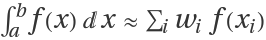.
The abscissas may or may not include the endpoints a and b of the interval. The option "QuadratureType" is used to control whether endpoints are included as abscissas.
With the default setting "QuadratureType"Closed, the endpoints are included as abscissas.
With the setting "QuadratureType"Open, the endpoints are not included as abscissas. In this case, the initial abscissa is chosen to be a half step above the lower endpoint and the final is a half step below the upper endpoint.

## Examples

### Basic Examples (1)

Find the Newton-Cotes value weight:

 In:=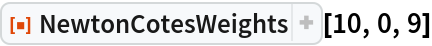Out=### Options (1)

Set "QuadratureType"Open to exclude the endpoints:

 In:=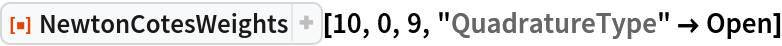Out=### Applications (5)

#### Approximate the area below a curve f(x)

Define the function:

 In:=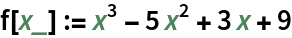Visualize the area between 0 and 5:

 In:=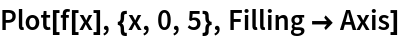Out=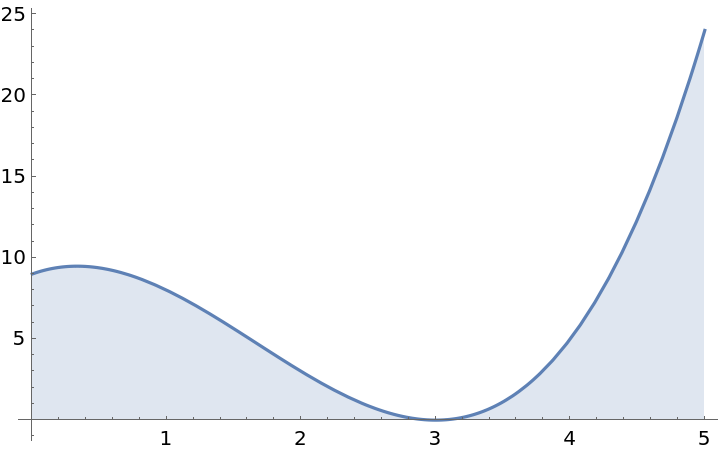Determine the Newton-Cotes weights:

 In:=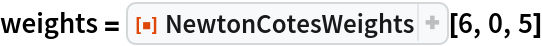Out=Multiply the value at each x-value by its weight:

 In:=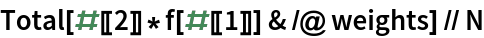Out=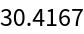Compare to the solution of NIntegrate:

 In:=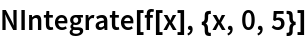Out=### Properties and Relations (1)

The resource function NewtonCotesError can be used to determine the error in the Newton–Cotes approximation:

 In:=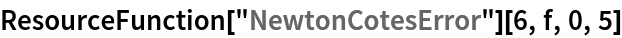Out=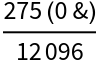## Version History

• 1.1.0 – 04 January 2021
• 1.0.0 – 20 November 2019